# The MCMC Procedure

### Matrix Functions in PROC MCMC

Subsections:

The MCMC procedure provides you with a number of CALL routines for performing simple matrix operations on declared arrays. With the exception of FILLMATRIX, IDENTITY, and ZEROMATRIX, the CALL routines listed in Table 61.44 do not support matrices or arrays that contain missing values.

Table 61.44: Matrix Functions in PROC MCMC

CALL Routine

Description

Performs an element-wise addition of two matrices or of a matrix and a scalar.

Calculates the Cholesky decomposition for a particular symmetric matrix.

Calculates the determinant of a specified matrix, which must be square.

Performs an element-wise multiplication of two matrices.

Replaces all of the element values of the input matrix with the specified value. You can use this routine with multidimensional numeric arrays.

Converts the input matrix to an identity matrix. Diagonal element values of the matrix are set to 1, and the rest of the values are set to 0.

Calculates a matrix that is the inverse of the input matrix. The input matrix must be a square, nonsingular matrix.

Calculates the matrix product of two input matrices.

Performs an element-wide subtraction of two matrices or of a matrix and a scalar.

Returns the transpose of a matrix.

Replaces all of the element values of the numeric input matrix with 0.

#### ADDMATRIX CALL Routine

The ADDMATRIX CALL routine performs an element-wise addition of two matrices or of a matrix and a scalar.

The syntax of the ADDMATRIX CALL routine is

• CALL ADDMATRIX (X, Y, Z);

where

• X specifies a scalar or an input matrix with dimensions(that is, X[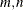])

• Y specifies a scalar or an input matrix with dimensions(that is, Y[])

• Z specifies an output matrix with dimensions(that is, Z[])

such that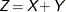#### CHOL CALL Routine

The CHOL CALL routine calculates the Cholesky decomposition for a particular symmetric matrix.

The syntax of the CHOL CALL routine is

• CALL CHOL (X, Y <, validate>);

where

• X specifies a symmetric positive-definite input matrix with dimensions(that is, X[m, m])

• Y is a variable that contains the Cholesky decomposition and specifies an output matrix with dimensions(that is, Y[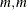])

• validate specifies an optional argument that can increase the processing speed by avoiding error checking:

• If validate = 0 or is not specified, then the matrix X is checked for symmetry.

• If validate = 1, then the matrix X is assumed to be symmetric.

such that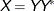where Y is a lower triangular matrix with strictly positive diagonal entries and Ydenotes the conjugate transpose of Y.

Both input and output matrices must be square and have the same dimensions. If X is symmetric positive-definite, Y is a lower triangle matrix. If X is not symmetric positive-definite, Y is filled with missing values.

#### DET CALL Routine

The determinant, the product of the eigenvalues, is a single numeric value. If the determinant of a matrix is zero, then that matrix is singular (that is, it does not have an inverse). The routine performs an LU decomposition and collects the product of the diagonals.

The syntax of the DET CALL routine is

• CALL DET (X, a);

where

• X specifies an input matrix with dimensions(that is, X[])

• a specifies the returned determinate value

such that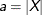#### ELEMMULT CALL Routine

The ELEMMULT CALL routine performs an element-wise multiplication of two matrices.

The syntax of the ELEMMULT CALL routine is

• CALL ELEMMULT (X, Y, Z);

where

• X specifies an input matrix with dimensions(that is, X[])

• Y specifies an input matrix with dimensions(that is, Y[])

• Z specifies an output matrix with dimensions(that is, Z[])

#### FILLMATRIX CALL Routine

The FILLMATRIX CALL routine replaces all of the element values of the input matrix with the specified value. You can use the FILLMATRIX CALL routine with multidimensional numeric arrays.

The syntax of the FILLMATRIX CALL routine is

• CALL FILLMATRIX (X, Y);

where

• X specifies an input numeric matrix

• Y specifies the numeric value that is used to fill the matrix

#### IDENTITY CALL Routine

The IDENTITY CALL routine converts the input matrix to an identity matrix. Diagonal element values of the matrix are set to 1, and the rest of the values are set to 0.

The syntax of the IDENTITY CALL routine is

• CALL IDENTITY (X);

where

• X specifies an input matrix with dimensions(that is, X[])

#### INV CALL Routine

The INV CALL routine calculates a matrix that is the inverse of the input matrix. The input matrix must be a square, nonsingular matrix.

The syntax of the INV CALL routine is

• CALL INV (X, Y);

where

• X specifies an input matrix with dimensions(that is, X[])

• Y specifies an output matrix with dimensions(that is, Y[])

#### MULT CALL Routine

The MULT CALL routine calculates the matrix product of two input matrices.

The syntax of the MULT CALL routine is

• CALL MULT (X, Y, Z);

where

• X specifies an input matrix with dimensions(that is, X[])

• Y specifies an input matrix with dimensions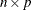(that is, Y[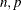])

• Z specifies an output matrix with dimensions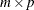(that is, Z[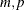])

The number of columns for the first input matrix must be the same as the number of rows for the second matrix. The calculated matrix is the last argument.

#### SUBTRACTMATRIX CALL Routine

The SUBTRACTMATRIX CALL routine performs an element-wide subtraction of two matrices or of a matrix and a scalar.

The syntax of the SUBTRACTMATRIX CALL routine is

• CALL SUBTRACTMATRIX (X, Y, Z);

where

• X specifies a scalar or an input matrix with dimensions(that is, X[])

• Y specifies a scalar or an input matrix with dimensions(that is, Y[])

• Z specifies an output matrix with dimensions(that is, Z[])

such that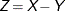#### TRANSPOSE CALL Routine

The TRANSPOSE CALL routine returns the transpose of a matrix.

The syntax of the TRANSPOSE CALL routine is

• CALL TRANSPOSE (X, Y);

where

• X specifies an input matrix with dimensions(that is, X[])

• Y specifies an output matrix with dimensions(that is, Y[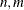])

#### ZEROMATRIX CALL Routine

The ZEROMATRIX CALL routine replaces all of the element values of the numeric input matrix with 0. You can use the ZEROMATRIX CALL routine with multidimensional numeric arrays.

The syntax of the ZEROMATRIX CALL routine is

• CALL ZEROMATRIX (X);

where

• X specifies a numeric input matrix.